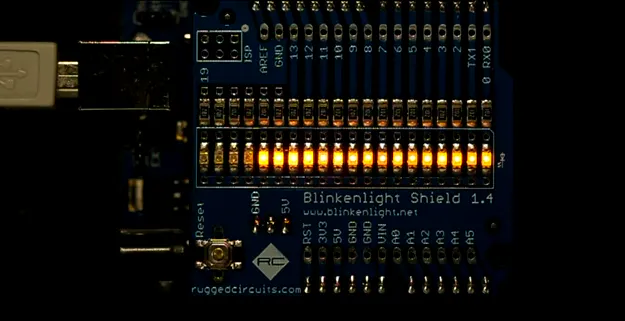Published

# Fast Counter

How fast can you toggle an IO pin?

Full instructions provided3,377## Things used in this project

### Hardware componentsArduino UNO & Genuino UNO
×1

## Code

### arduino_pins___avr_ports.c

C/C++
arduino_pins___avr_ports.c
```//
//
//
//  This program is free software: you can redistribute it and/or modify
//  the Free Software Foundation, either version 3 of the License, or
//  (at your option) any later version.
//
//  This program is distributed in the hope that it will be useful,
//  but WITHOUT ANY WARRANTY; without even the implied warranty of
//  MERCHANTABILITY or FITNESS FOR A PARTICULAR PURPOSE. See the
//  GNU General Public License for more details.
//
//  You should have received a copy of the GNU General Public License
//  along with this program. If not, see http://www.gnu.org/licenses/

const uint8_t bit_0 = 1<<0;
const uint8_t bit_1 = 1<<1;
const uint8_t bit_2 = 1<<2;
const uint8_t bit_3 = 1<<3;
const uint8_t bit_4 = 1<<4;
const uint8_t bit_5 = 1<<5;
const uint8_t bit_6 = 1<<6;
const uint8_t bit_7 = 1<<7;

#define COUNT_1                PIND = bit_0;
#define COUNT_3    COUNT_1;    PIND = bit_1; COUNT_1;
#define COUNT_7    COUNT_3;    PIND = bit_2; COUNT_3;
#define COUNT_15   COUNT_7;    PIND = bit_3; COUNT_7;
#define COUNT_31   COUNT_15;   PIND = bit_4; COUNT_15;
#define COUNT_63   COUNT_31;   PIND = bit_5; COUNT_31;
#define COUNT_127  COUNT_63;   PIND = bit_6; COUNT_63;
#define COUNT_255  COUNT_127;  PIND = bit_7; COUNT_127;
#define COUNT_511  COUNT_255;  PINB = bit_0; COUNT_255;
#define COUNT_1023 COUNT_511;  PINB = bit_1; COUNT_511;
#define COUNT_2047 COUNT_1023; PINB = bit_2; COUNT_1023;
#define COUNT_4095 COUNT_2047; PINB = bit_3; COUNT_2047;

void count_8191() { COUNT_4095; PINB = bit_4; COUNT_4095; }

#define COUNT_16383  count_8191(); PINB = bit_5; count_8191();
#define COUNT_32767  COUNT_16383;  PINC = bit_0; COUNT_16383;
#define COUNT_65535  COUNT_32767;  PINC = bit_1; COUNT_32767;
#define COUNT_131071 COUNT_65535;  PINC = bit_2; COUNT_65535;
#define COUNT_262143 COUNT_131071; PINC = bit_3; COUNT_131071;
#define COUNT_524287 COUNT_262143; PINC = bit_4; COUNT_262143;
#define COUNT_524288 COUNT_524287; PINC = bit_5;

#define TWICE(a) a a

void setup() {
DDRD = 0b11111111; // set digital  0- 7 to output
DDRB = 0b00111111; // set digital  8-13 to output
DDRC = 0b00111111; // set digital 14-19 to output (coincidences with analog 0-5)

// ensure we do not get interrupted during prescaler manipulation
cli();

/*
// activate this block by removing the previous comment
// set clock prescaler as desired to slow down the timer
const uint8_t clock_prescaler_1   = 0;
const uint8_t clock_prescaler_2   = 1;
const uint8_t clock_prescaler_4   = 2;
const uint8_t clock_prescaler_8   = 3;
const uint8_t clock_prescaler_16  = 4;
const uint8_t clock_prescaler_32  = 5;
const uint8_t clock_prescaler_64  = 6;
const uint8_t clock_prescaler_128 = 7;
const uint8_t clock_prescaler_256 = 8;

// prepare to set clock prescaler: write CLKPCE bit to one and all the other to zero
CLKPR = 1<<CLKPCE;
// set clock prescaler immediately after preparing to do so
CLKPR = clock_prescaler_256;
/**/
// do not enable interrupts again because we do not want
// interrupts to slow us any down
}

void loop() {
l0:
TWICE(TWICE(TWICE(TWICE(TWICE(COUNT_524288)))));
goto l0;
}
```